# chapter 9 - Force and laws of motion notes

Welcome to Class 9 force and laws of Motion Notes for Chapter 9.The topics in this page are balanced and unbalanced forces ,Laws of motion,First law of motion, Momentum, Second Law of motion ,Third Law of motion and Momentum Conservation.This is according to CBSE and the NCERT textbook. If you like the study material, feel free to share the link as much as possible.

Table Of Content

In previous chapter motion class 9 we already learned about motion and variables of motion. You must have noticed that in that chapter we only analyzed motion using variables like speed, velocity, acceleration etc. Here in this i.e., force and laws of motion we will get to know what causes motion. In this page find easy to learn and understand ,class 9 science, chapter 9 - Force and laws of motion notes.

## Introduction

• While studying kinematics, we have already studied about the position, distance and displacement, and acceleration of a moving particle.
• Here in this chapter, we would take our understanding one step further to learn about origins of acceleration or force.
• Here we will specifically consider the cause behind the moving objects i.e. what causes the objects to move.
• Thus we will learn the theory of motion based on the ideas of mass and force and the laws connecting these physical concepts to the kinematics quantities.
• Concept of force is central to all of physics whether it is classical physics, nuclear physics, quantum physics or any other form of physics
• So what is force? When we push or pull anybody we are said to exert force on the body
• Push or pull applied on a body does not exactly define the force in general. We can define force as an influence causing a body at rest or moving with constant velocity to undergo acceleration
• There are many ways in which one body can exert force on another body. Few examples are given below
(a) Stretched springs exerts force on the bodies attached to its ends
(b) Compressed air in a container exerts force on the walls of the container
(c) Force can be used to deform a flexible object
• Force of gravitational attraction exerted by earth is a kind of force that acts on every physical body on the earth and is called the weight of the body

## Balanced and Unbalanced forces

• There are two types of forces namely balanced forces and unbalanced forces

### (a) Balanced Forces

• If the resultant of all forces acting on a body is zero then the forces are called balanced forces.
• To understand this concept consider an object rests on a surface such as a block on the table as shown below in the figure 1. Weight of the block is balanced by the reaction force from the table. The table pushes up against the block.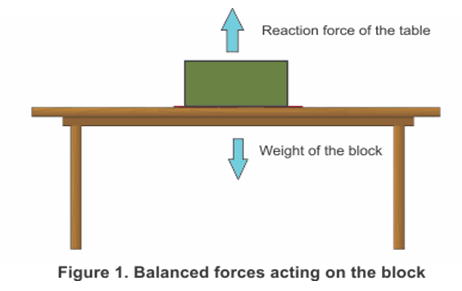So the weight of a block lying on a table is balanced by the reaction force from the table top
• If the forces on an object are balanced (or if there are no forces acting on it) than the object that is not moving stays still and the object that is moving continues to move at the same speed and in the same direction.
• Although balanced forces cannot produce motion in a stationary body or stop a moving body but they can however change the shape of the body.

### (b) Unbalanced Forces

• If the resultant forces acting on a body is not zero the forces are called unbalanced forces.
• To understand this consider the figure 2 which shows a block on a horizontal table and two strings X and Y are tied to the two opposite faces of the block.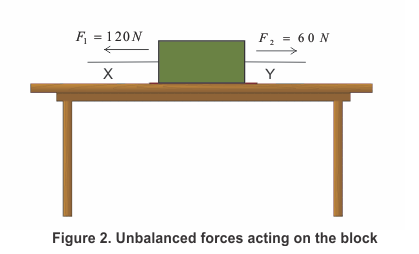• Let us now pull the block using this string in two different directions such that two opposite forces of different magnitudes acts on the block.
• Since two forces acting on the block are of different magnitude the block would begin to move in the direction of the greater force.
• Thus, the two forces acting on the block are not balanced and the unbalanced force acts in the direction the block moves.
• So unbalanced forces can move a stationary body and they can stop a moving body.
• The size of the overall force acting on an object is called the resultant force. If the forces are balanced, this is zero. In the example above, the resultant force is the difference between the two forces F1 and F2, which is 120 - 60 = 60 N.
• If all the forces acting on a body result in an unbalanced force, then the unbalanced force can accelerate the body. It means that a net force or resulting force acting on a body can either change the magnitude of its velocity or change the direction of its velocity.
• The force that opposes the relative motion between the surfaces of two objects in contact and acts along the surfaces in contact is called the force of friction or simply friction.

## Laws of motion

• Newton gave three laws of motion that describe the motion of bodies. These laws are known as Newton's Laws of motion.
• They describe the relationship between the forces acting on a body and its motion due to those forces.
• The three laws of motion were first compiled by Sir Isaac Newton in his work Principia Mathematica, first published in 1687. Newton used these laws to explain and investigate the motion of many physical objects and systems.
• We shall now learn about Newton's First law of motion

### 4. Newton's First Law of motion

• The first law of motion is stated as:
An object remains in a state of rest or of uniform motion in a straight line unless compelled to change that state by an applied force.
• All objects resist a change in their state of motion. The tendency of undisturbed objects whether they are at rest or moving with uniform velocity is called inertia. Hence, the first law of motion is also known as the law of inertia.
• Greater the inertia of the body greater will be the force required to bring the change in the state of rest or uniform motion of the body.
• Mass is the measure of the inertia of the body so heavier objects have more inertia then lighter objects. For example a ball of 2Kg has more inertia then a football and it takes more effort to kick a 2Kg ball then it takes to kick a foot ball.
• Another example is even a small child can push a toy car. However, An adult also can't push a loaded vehicle forward.
• Newton's first law of motion gives us a definition of force. It says that
Force is something that changes or tends to change the state of rest or uniform motion of a body

### Momentum

• Before discussing about second law of motion we shall first learn about momentum of a moving object.
• From our daily life experiences like during the game of table tennis if the ball hits a player it does not hurt him. On the other hand, when a fast moving cricket ball hits a spectator, it may hurt him.
• This suggests that impact produced by moving objects depends on both their mass and velocity.
• So, there appears to exist some quantity of importance that combines the object's mass and its velocity called momentum and was introduced by Newton.
• Momentum can be defined as "mass in motion". All objects have mass; so if an object is moving, then it has momentum - it has its mass in motion.
• The momentum, p of an object is defined as the product of its mass, $m$ and velocity, $v$. That is,
momentum p=mv           (1)
• Momentum has both direction and magnitude so it is a vector quantity. Its direction is the same as that of velocity, $v$.
• The SI unit of momentum is kilogram-meter per second (kg m s-1).
• Since the application of an unbalanced force brings a change in the velocity of the object, it is therefore clear that a force also produces a change of momentum.
• We define the momentum at the start of the time interval is the initial momentum and at the end of the time interval is the final momentum.
• When the object moves then it gains momentum as the velocity increases. Hence greater the velocity greater is the momentum.

### 6. Second Law of motion

• Newton's second law of motion gives the relationship between the force and acceleration.
• The second law of motion states that
the rate of change of momentum of an object is proportional to the applied unbalanced force in the direction of force
• The rate of change of momentum of an object is proportional to the applied force. So, Newton's second law of motion can be expressed as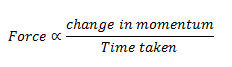• Suppose an object of mass, $m$ is moving along a straight line with an initial velocity, $u$. It is uniformly accelerated to velocity, $v$ in time, $t$ by the application of a constant force, $F$ throughout the time, $t$.
• The initial and final momentum of the object will be, p1 = mu and p2 = mv respectively.
• Now change in momentum would be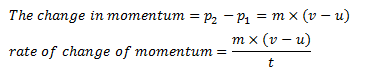• Now force applied is proportional to rate of change of momentum. So,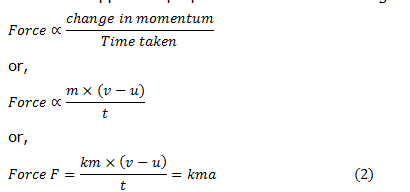where, a =(v-u)/t is the acceleration which is the rate of change of velocity. The quantity,k is a constant of proportionality
• The unit of force is chosen in such a way that the value of the constant, k becomes one.
• For this, one unit of force is defined as the amount that produces an acceleration of 1 ms-2 in an object of 1 kg mass. That is,
1 unit of force = k × (1 kg) × (1 m s-2). Thus, the value of k becomes 1.
• From Eq. 2
F = ma           (3)
• The unit of force is kgms-2 or Newton, which has the symbol N.
• The second law of motion gives us a method to measure the force acting on an object as a product of its mass and acceleration.

### 7. Third Law of motion

• Newton's third law of motion states that
Whenever a body exerts a force on another body, the second body exerts an equal and opposite force on the first body
• So according to third law of motion to every action there is an equal and opposite reaction. This tells us that all forces in nature acts in pairs.
• These two forces are always equal in magnitude but opposite in direction.
• These forces act on different objects and never on the same object.
• Few applications of third law of motion are
1. If you throw a heavy ball or block away from your body, the force exerted will push back on you, possibly pushing you backward onto the ground.
2. A book lying on a table exerts a force on the table which is equal to the weight of the book (action force). The table supports the book, by exerting an equal force on the book (reaction). As the system is at rest, net force on it is zero. Therefore, forces of action and reaction must be equal and opposite.
3. When a gun is fired, the bullet moves forward (action). The gun recoils backwards (reaction).

## Law of conservation of momentum

• Law of conservation of linear momentum is a extremely important consequence of Newton's third law of motion in combination with the second law of motion.
• According to law of conservation of momentum
When two or more bodies acts upon each other their total momentum remains constant provided no external forces are acting
• So, Momentum is never created or destroyed.
• When this law is applied for a collision between two bodies, the total momentum of the colliding bodies before collision is equal to the total momentum after collision.
• We can apply this law for a collision between two vehicles. This law is applicable for all types of collisions.
• Consider two particles say A and B of mass m1 and m2 collide with each other and forces acting on these particles are only the ones they exert on each other.
• Let u1 and v1 be the initial and final velocities of particle A and similarly, u2 and v2for particle B. Let the two particles be in contact for a time t. So, Change in momentum of A=m1 (v1-u1) Change in momentum of B=m2 (v2-u2 )
• During the collision, let A impart an average force equal to FBA on B and let B exert an average FAB on A. We know that from third law of motion FBA=-FAB            (4)
Here,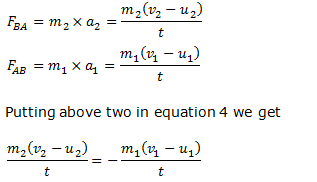canceling t on both sides and rearranging the equation we get
${m_1}{u_1} + {m_2}{u_2} = {m_1}{v_1} + {m_2}{v_2}$              (5)
Now, ${m_1}{u_1} + {m_2}{u_2}$ represents the total momentum of particles A and B before collision and ${m_1}{v_1} + {m_2}{v_2}$ represents the total momentum of particles after collision. This means that
Total momentum before collision=total momentum after collision
• Equation 5 which ${m_1}{u_1} + {m_2}{u_2} = {m_1}{v_1} + {m_2}{v_2}$ ,is known as the law of conservation of momentum.
• Thus we conclude that when two particles are subjected only to their mutual interactions ,the sum of the momentums of the bodies remains constant in time or we can say the total momentum of the two particles does not change because of the any mutual interactions between them.
• For any kind of force between two particles then sum of the momentum ,both before and after the action of force should be equal i.e total momentum remains constant.
• Law of conservation of linear momentum is one of the most fundamental and important principle of mechanics.
• Once again ,the total momentum of two or any number of particles of interacting particles is constant if they are isolated from outside influences (or no resultant external forces is acting on the particles).

## Summary

Here is the Class 9 Force and Laws of Motion notes Summary
• An object continues to be in a state of rest or of uniform motion along a straight line unless acted upon by an unbalanced force. This is called First law of Motion
• The rate of change of momentum of an object is proportional to the applied unbalanced force in the direction of the force.This is called Second law of Motion
• To every action, there is an equal and opposite reaction and they act on two different bodies.This is called Third law of Motion
• Momentum is defined as product of mass and velocity
• The total momentum remains conserved if there is no external force. This is called law of conservation of momentum